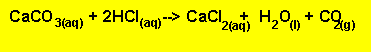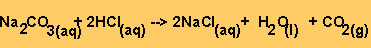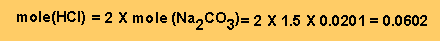Solution

Step 1 - Work out the equations for all the reactions taking place.

The equation for the reaction between HCl with the limestone is given below.
Equation 1The equation for the reaction between HCl and sodium carbonate is given below.
Equation 2Step 2 - From equation 2 we can work out the mole of HCl left unreacted.Step 3 - Work out how much HCl did react. Subtract the amount in excess from the amount originally added.

Amount originally added - amount in excess = amount reacted.
(0.1 X 1.1) - 0.0602 = 0.0498 mole of HCl reacted.

Step 3 - Using equation 1 calculate the amount of caclium carbonate present.
Mole of HCl /2 = mole of calcium carbonate = 0.0498/2 = 0.0249 mole.

Step 4 - Calculate the mass of calcium carbonate present.

0.0249 X formula mass = 0.0249 X 100 = 2.49 grams

Step 4 Calculate the percentage
2.49/3.00 X100 = 83.0%